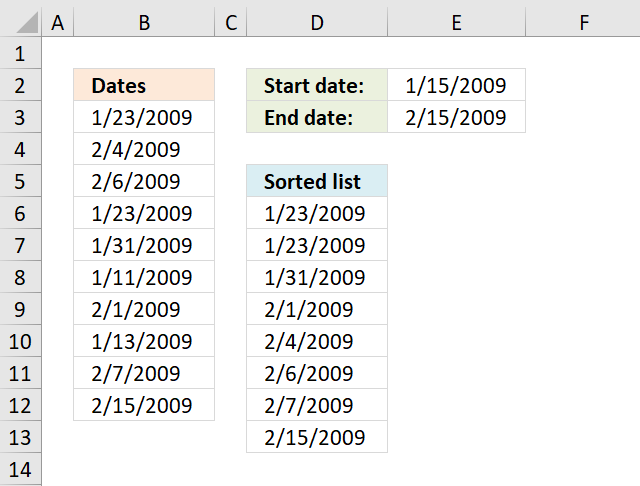Author: Oscar Cronquist Article last updated on November 19, 2018Array formula in D5:

=SMALL(IF((\$B\$3:\$B\$12<=\$E\$3)*(\$B\$3:\$B\$12>=\$E\$2), \$B\$3:\$B\$12, "A"), ROWS(\$A\$1:A1))

How to create an array formula

1. Copy array formula
2. Select cell D5
3. Paste formula in formula bar
4. Press and hold Ctrl + Shift
5. Press Enter

How to copy array formula

1. Select cell D5
2. Copy (Ctrl + c)
3. Select cell range D6:D15
4. Paste (Ctrl + v)

Explaining formula in cell D5

Step 1 - Comparison end date

The less than sign and the equal sig allows you to check if values in cell range are less than or equal to the end date.

(\$B\$3:\$B\$12<=\$E\$3)

becomes

{39836;39848;39850;39836;39844;39824;39845;39826;39851;39859}<=39859

and returns

{TRUE; TRUE;TRUE; TRUE;TRUE; TRUE;TRUE; TRUE;TRUE; TRUE}.

Step 2 - Comparison start date

The greater than sign and the equal sig allows you to check if values in cell range are greater than or equal to the start date.

(\$B\$3:\$B\$12>=\$E\$2)

becomes

{39836;39848;39850;39836;39844;39824;39845;39826;39851;39859}>=39828

and returns

{TRUE;TRUE; TRUE; TRUE;TRUE;FALSE;TRUE; FALSE;TRUE; TRUE}

Step 3 - Multiply arrays

Both conditions must evaluate to TRUE.

(\$B\$3:\$B\$12<=\$E\$3)*(\$B\$3:\$B\$12>=\$E\$2)

becomes

{TRUE; TRUE;TRUE; TRUE;TRUE; TRUE;TRUE; TRUE;TRUE; TRUE}*{TRUE;TRUE; TRUE; TRUE;TRUE;FALSE;TRUE; FALSE;TRUE; TRUE}

and returns

{1;1;1;1;1;0;1;0;1;1}

 Boolean Boolean Multiply FALSE FALSE 0 TRUE FALSE 0 TRUE TRUE 1

Step 4 - Replace TRUE with the corresponding date

The IF function replaces TRUE values with the corresponding dates and FALSE with "A".

IF((\$B\$3:\$B\$12<=\$E\$3)*(\$B\$3:\$B\$12>=\$E\$2), \$B\$3:\$B\$12, "A")

becomes

IF({1;1;1;1;1;0;1;0;1;1}, {39836;39848;39850;39836;39844;39824;39845;39826;39851;39859}, "A")

and returns

{39836; 39848; 39850; 39836; 39844; "A"; 39845; "A"; 39851; 39859}

Step 5 - Sort numbers (dates)

The SMALL function extracts the k-th smallest number and ignores text values like "A".

SMALL(IF((\$B\$3:\$B\$12<=\$E\$3)*(\$B\$3:\$B\$12>=\$E\$2), \$B\$3:\$B\$12, "A"), ROWS(\$A\$1:A1))

becomes

SMALL({39836; 39848; 39850; 39836; 39844; "A"; 39845; "A"; 39851; 39859}, ROWS(\$A\$1:A1))

The ROWS function counts rows in a cell reference, this cell reference \$A\$1:A1 expands as the formula is copied to cells below. This will extract a new value in each cell.

SMALL({39836; 39848; 39850; 39836; 39844; "A"; 39845; "A"; 39851; 39859}, ROWS(\$A\$1:A1))

becomes

SMALL({39836; 39848; 39850; 39836; 39844; "A"; 39845; "A"; 39851; 39859}, 1)

and returns "1/23/2009" in cell D6.

Get Excel *.xlsx file

sort-dates-within-a-date-range-using-excel-array-formula.xlsx